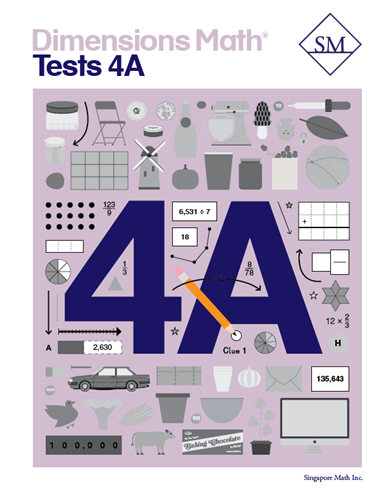# Dimensions Math Tests 4A

\$ 12.00

Chapter 1: Numbers to One Million
Test A
Test B
Test A
Test B
Chapter 3: Multiples and Factors
Test A
Test B
Chapter 4: Multiplication
Test A
Test B
Chapter 5: Division
Test A
Test B
Continual Assessment 1
Test A
Test B
Chapter 6: Fractions
Test A
Test B
Chapter 7: Adding and Subtracting Fractions
Test A
Test B
Chapter 8: Multiplying a Fraction and a Whole Number
Test A
Test B
Chapter 9: Line Graphs and Line Plots
Test A
Test B
Continual Assessment 2
Test A
Test B

Dimensions Math® PreK-5 series features the progression, rigor, and pacing that define Singapore math. Throughout the series, five characters offer students suggestions on how to think about problems. They remind students of strategies they’ve learned and point out important information that encourages them to come up with their own solutions.

Textbook lessons begin with a task that allows students to apply their previous knowledge and learn through discussion. Once students have mastered a concept with the use of concrete and pictorial aids, they are ready to take on more abstract mathematical problem sets. They reach fluency by collecting various strategies along the way and applying them to new problems. Word problems give students a sense of math in real-world contexts.

Workbooks offer independent practice that follows a careful progression of exercise variation. Each textbook lesson includes a corresponding workbook exercise that starts with pictorial representation and progresses to more challenging abstract problems. Workbooks for PreK-2 are perforated.

Teacher’s Guides include lesson plans, mathematical background, games, helpful suggestions, and comprehensive resources for daily lessons. Lessons are laid out clearly and activities are designed for the whole class, small groups, and extension.

ISBN  9781947226531